Past artworks that have been sold and are currently held in Private Collections, they are made available here for your reference only.##### Fox Amongst The Birches
Currency conversion from USD to: [convert number=505.00 from="usd" to="zar"] ZAR | [convert number=505.00 from="usd" to="gbp"] GBP | [convert number=505.00 from="usd" to="eur"] EUR     Medium: Oil and Silver Leaf on Canvas Size: 30cm by 30cm SOLD
\$ 505.00##### Magic Mushrooms
Currency conversion from USD to: [convert number=505.00 from="usd" to="zar"] ZAR | [convert number=505.00 from="usd" to="gbp"] GBP | [convert number=505.00 from="usd" to="eur"] EUR     Medium: Oil and Gold Leaf on Canvas Size: 30cm by 30cm SOLD
\$ 505.00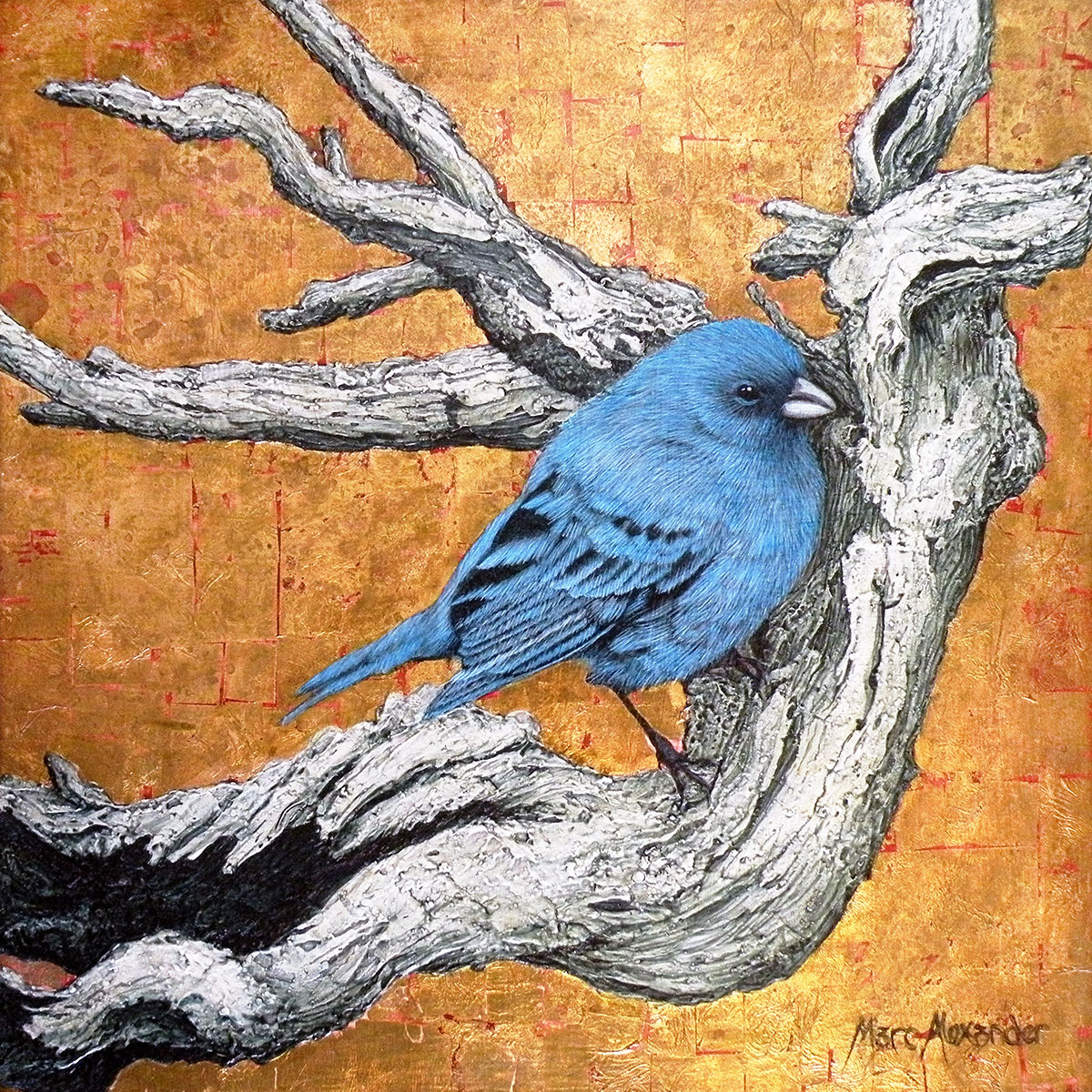##### Indigo Bunting
Currency conversion from USD to: [convert number=505.00 from="usd" to="zar"] ZAR | [convert number=505.00 from="usd" to="gbp"] GBP | [convert number=505.00 from="usd" to="eur"] EUR     Medium: Oil and Gold Leaf on Canvas Size: 30cm by 30cm SOLD
\$ 505.00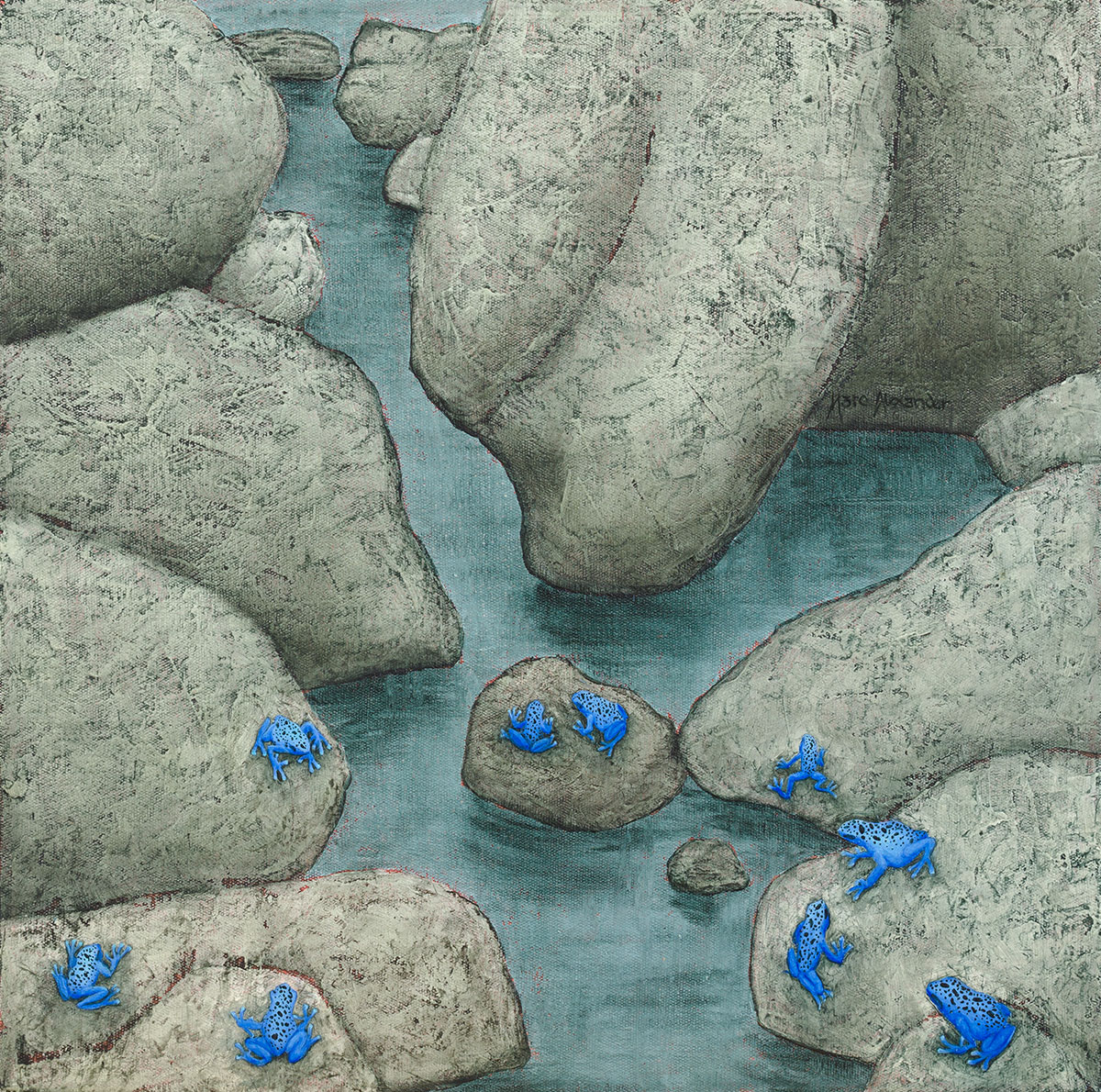##### Poison Arrow Frogs
Currency conversion from USD to: [convert number=505.00 from="usd" to="zar"] ZAR | [convert number=505.00 from="usd" to="gbp"] GBP | [convert number=505.00 from="usd" to="eur"] EUR     Medium: Oil on Canvas Size: 40cm by 40cm SOLD
\$ 505.00##### Rabbit Hole
Currency conversion from USD to: [convert number=505.00 from="usd" to="zar"] ZAR | [convert number=505.00 from="usd" to="gbp"] GBP | [convert number=505.00 from="usd" to="eur"] EUR     Medium: Oil and Gold Leaf on Canvas Size: 30cm by 30cm SOLD
\$ 505.00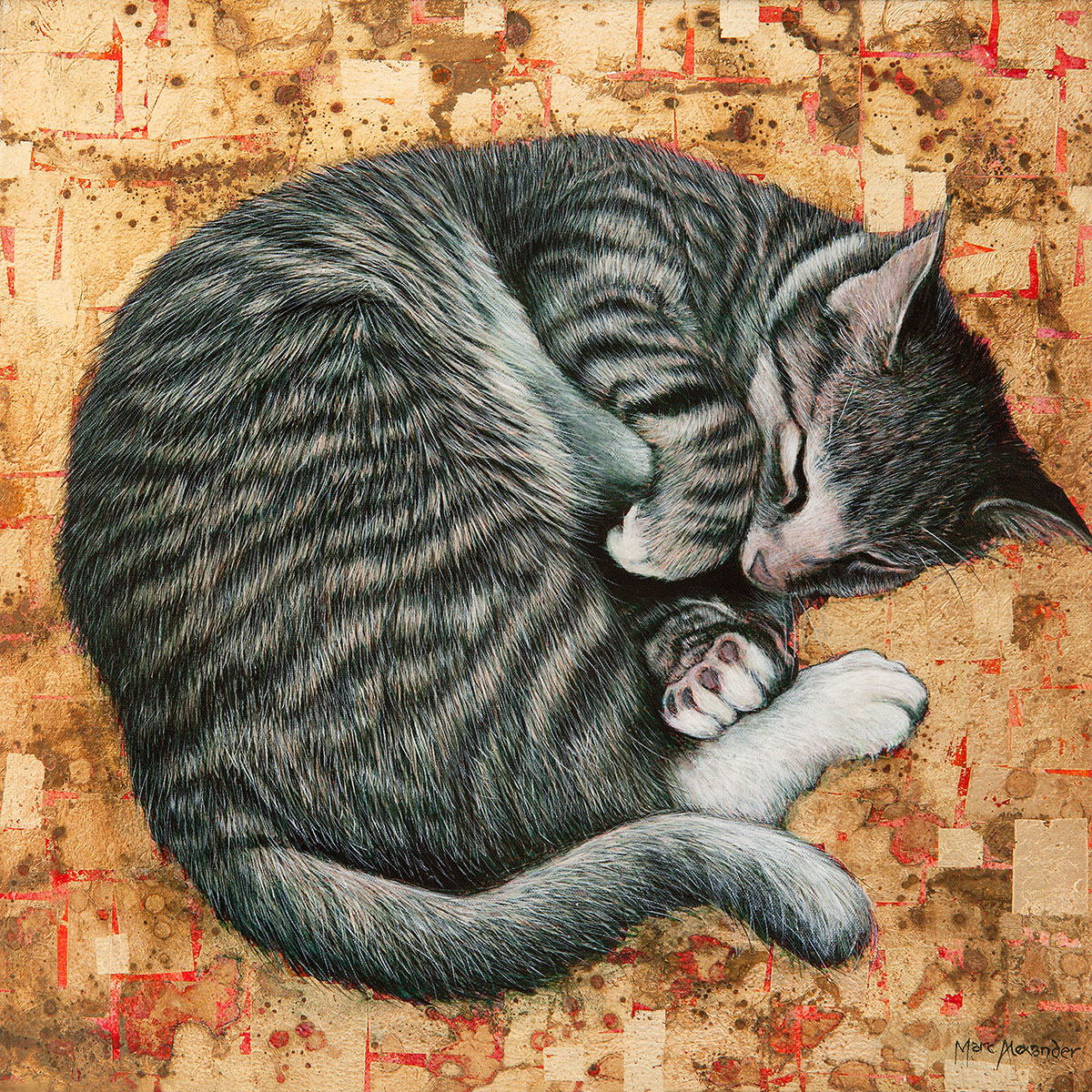##### Sleeping Cat
Currency conversion from USD to: [convert number=505.00 from="usd" to="zar"] ZAR | [convert number=505.00 from="usd" to="gbp"] GBP | [convert number=505.00 from="usd" to="eur"] EUR     Medium: Oil and Gold Leaf on Board Size: 30cm by 30cm SOLD
\$ 505.00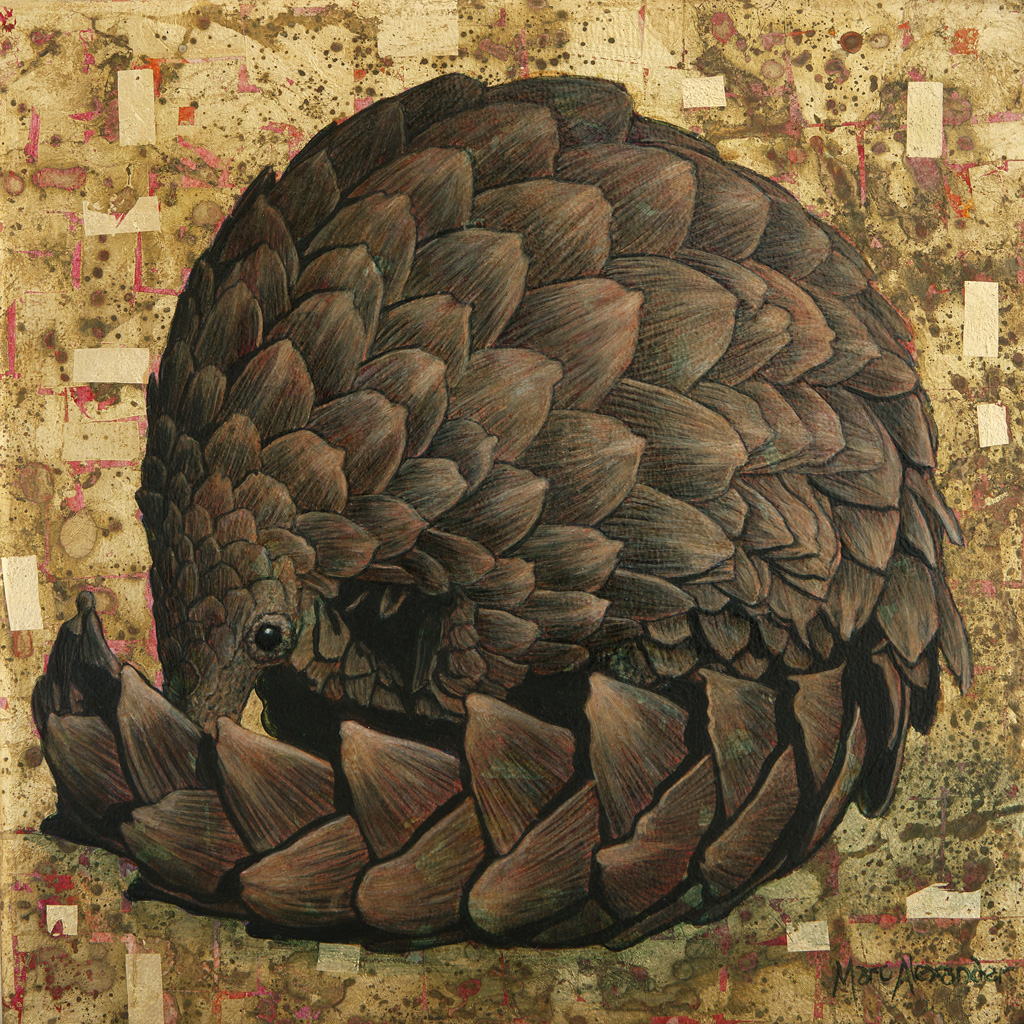##### Pangolin
Currency conversion from USD to: [convert number=650.00 from="usd" to="zar"] ZAR | [convert number=650.00 from="usd" to="gbp"] GBP | [convert number=650.00 from="usd" to="eur"] EUR     Medium: Oil and Gold Leaf on Canvas Size: 30cm by 30cm SOLD
\$ 650.00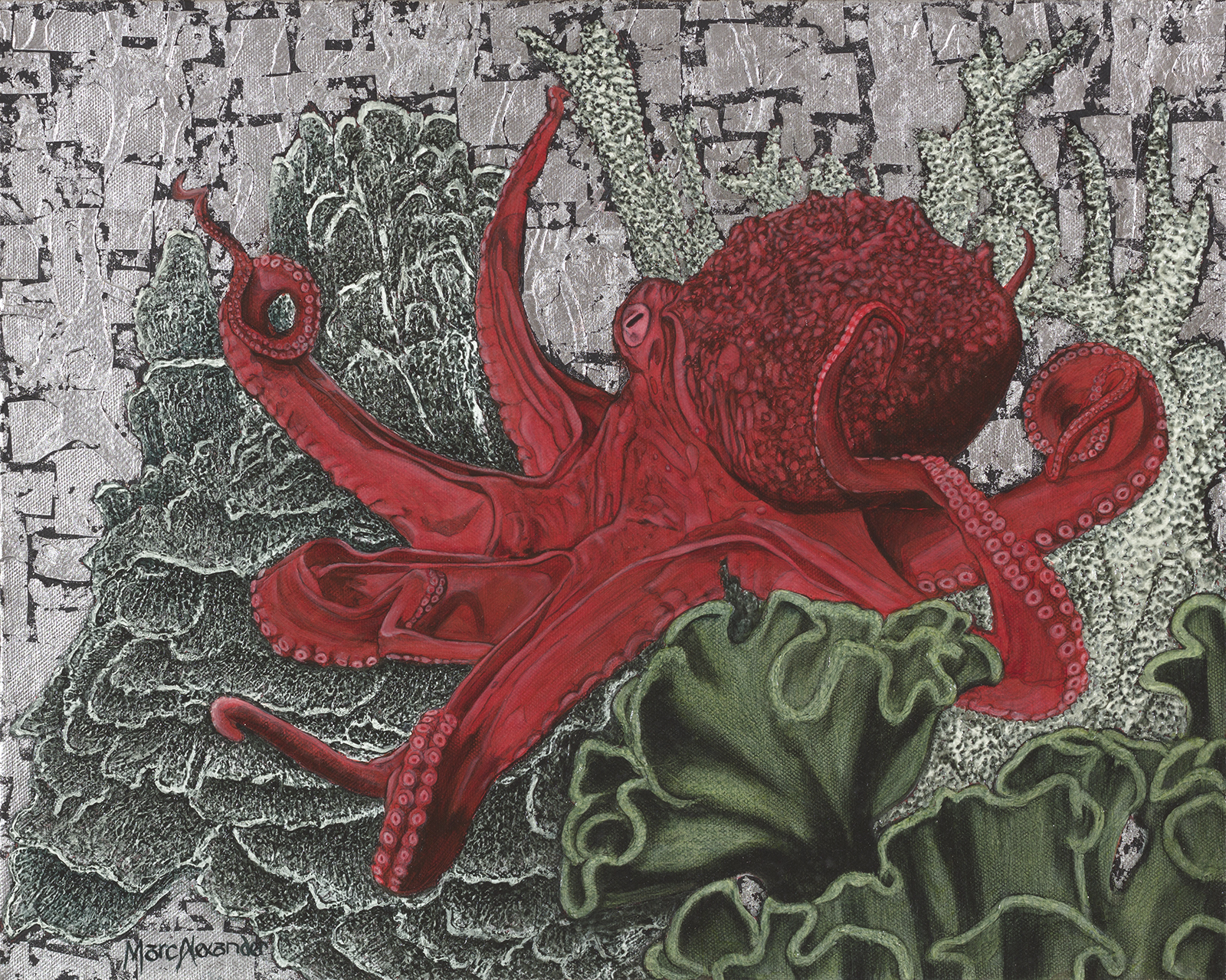##### Red Octopus
Currency conversion from USD to: [convert number=650.00 from="usd" to="zar"] ZAR | [convert number=650.00 from="usd" to="gbp"] GBP | [convert number=650.00 from="usd" to="eur"] EUR     Medium: Oil and Silver Leaf on Canvas Size: 40cm by 50cm SOLD
\$ 650.00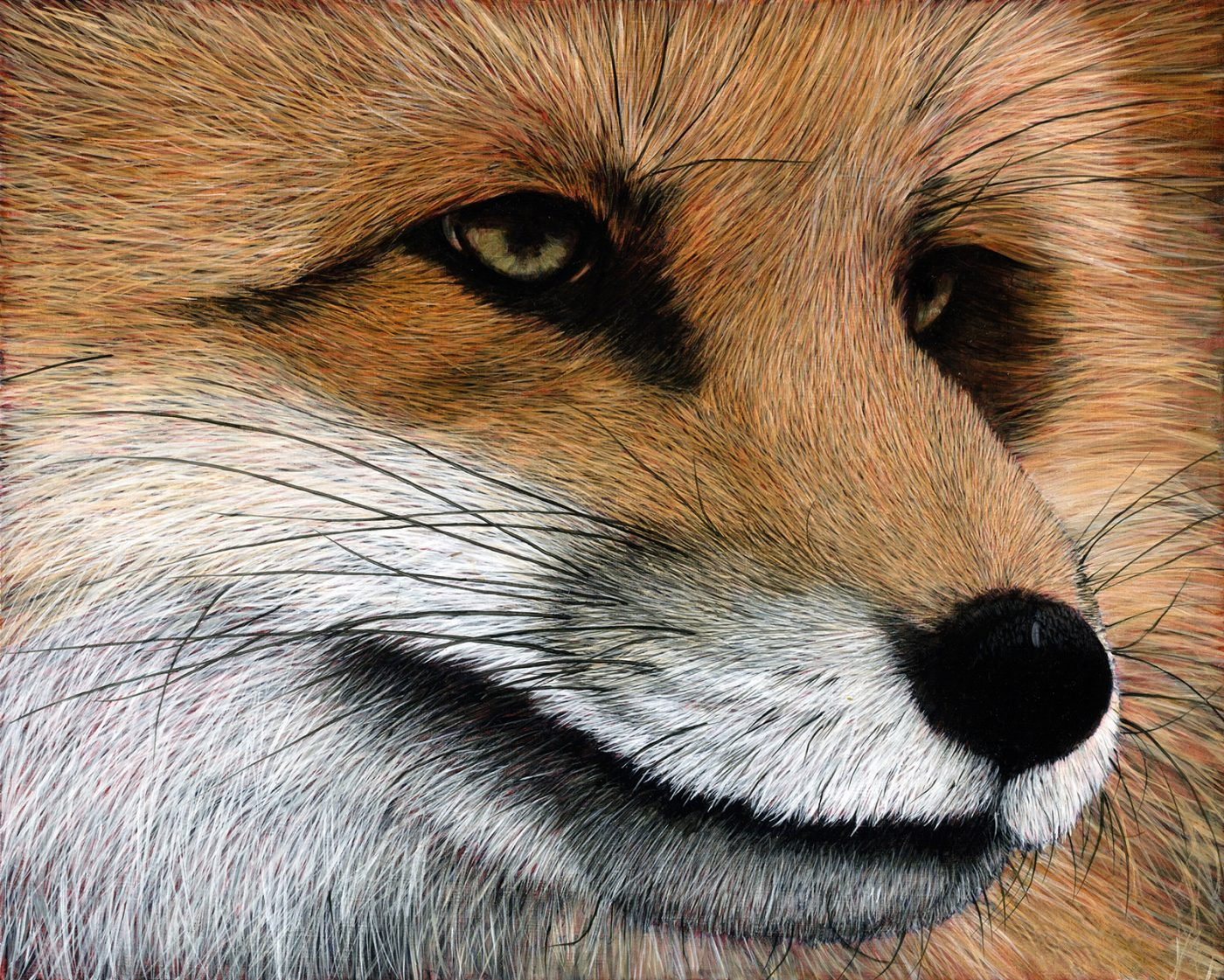##### Red Fox
Currency conversion from USD to: [convert number=650.00 from="usd" to="zar"] ZAR | [convert number=650.00 from="usd" to="gbp"] GBP | [convert number=650.00 from="usd" to="eur"] EUR     Medium: Oil on Canvas Size: 40cm by 50cm SOLD
\$ 650.00
Sale!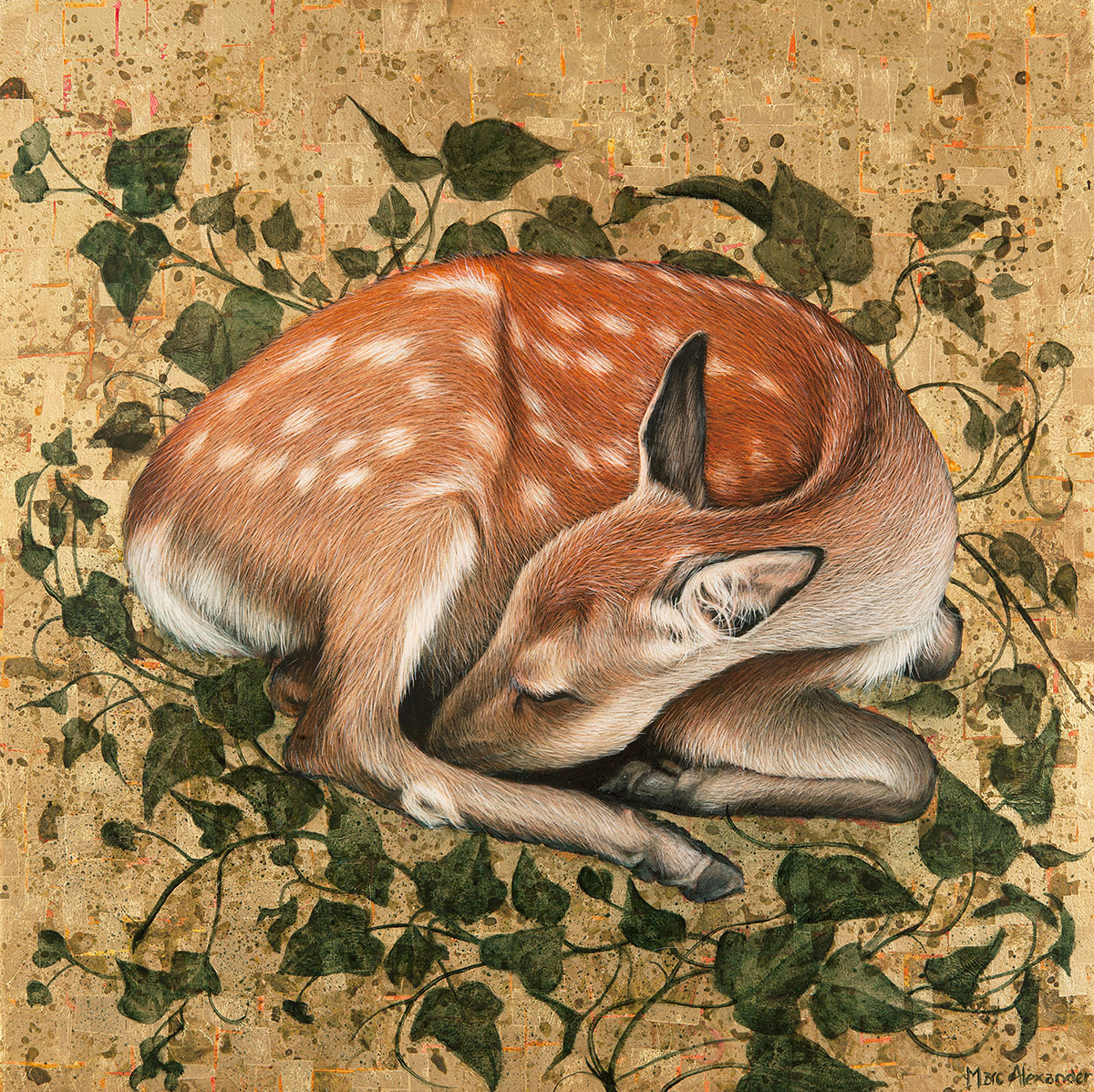##### Sleeping Fawn
Currency conversion from USD to: [convert number=820.00 from="usd" to="zar"] ZAR | [convert number=820.00 from="usd" to="gbp"] GBP | [convert number=820.00 from="usd" to="eur"] EUR   Medium: Oil and Gold Leaf on Board Size: 50cm by 50cm
\$ 1,400.00 \$ 820.00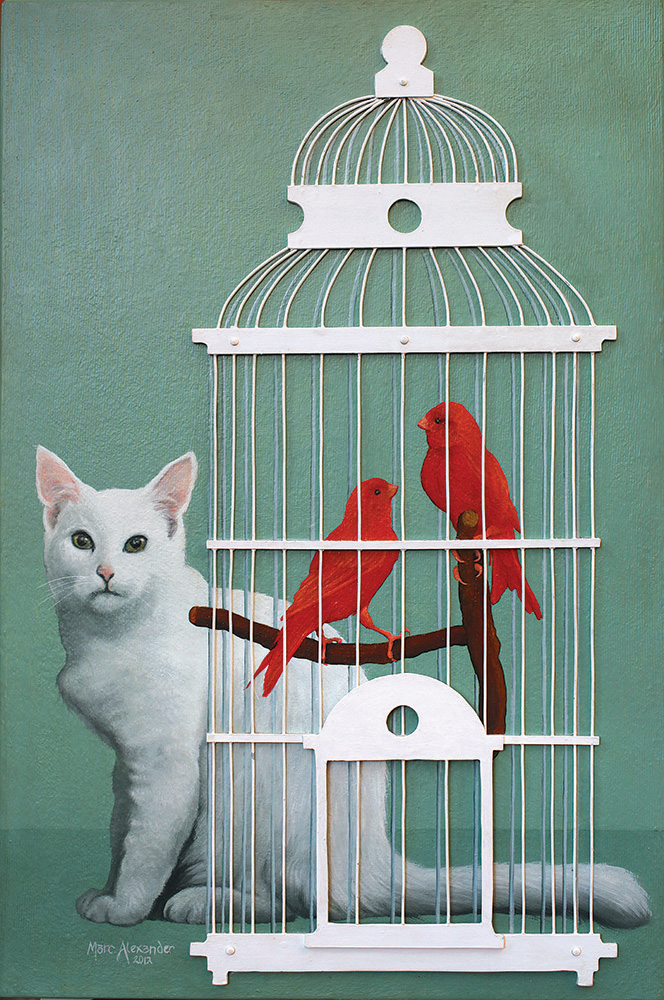##### Bird Cage I
Currency conversion from USD to: [convert number=1450.00 from="usd" to="zar"] ZAR | [convert number=1450.00 from="usd" to="gbp"] GBP | [convert number=1450.00 from="usd" to="eur"] EUR     Medium: Oil and wire on Canvas Size: 60cm by 40cm SOLD
\$ 1,050.00##### Snowy Owl
Currency conversion from USD to: [convert number=1400.00 from="usd" to="zar"] ZAR | [convert number=1400.00 from="usd" to="gbp"] GBP | [convert number=1400.00 from="usd" to="eur"] EUR     Medium: Oil and Gold Leaf on Canvas Size: 74cm by 50cm SOLD
\$ 1,400.00##### Crow’s Nest
Currency conversion from USD to: [convert number=1400.00 from="usd" to="zar"] ZAR | [convert number=1400.00 from="usd" to="gbp"] GBP | [convert number=1400.00 from="usd" to="eur"] EUR     Medium: Oil on Canvas Size: 40cm by 40cm SOLD
\$ 1,400.00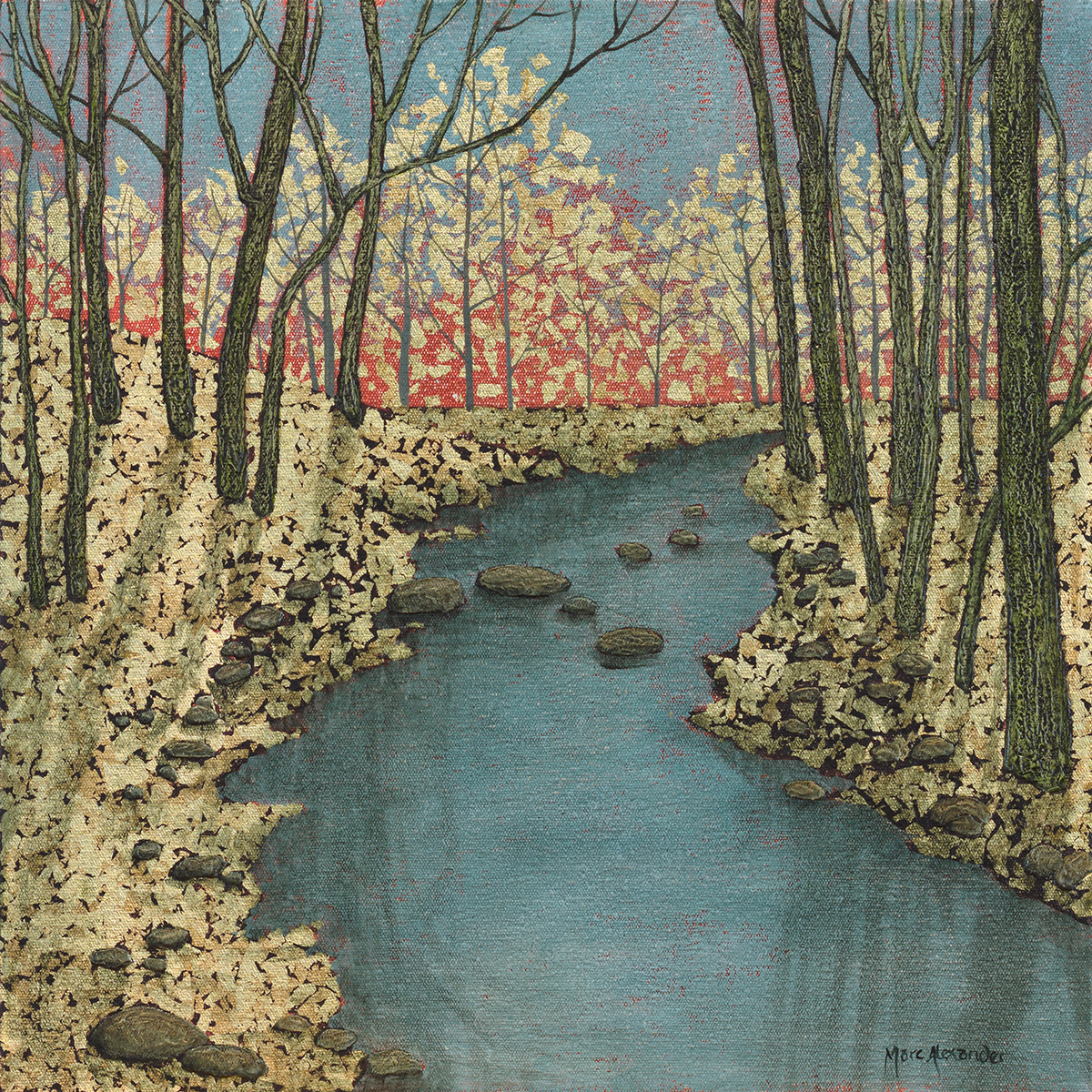##### Forest Stream I
Currency conversion from USD to: [convert number=1400.00 from="usd" to="zar"] ZAR | [convert number=1400.00 from="usd" to="gbp"] GBP | [convert number=1400.00 from="usd" to="eur"] EUR     Medium: Oil and Gold Leaf on Canvas Size: 40cm by 40cm SOLD
\$ 1,400.00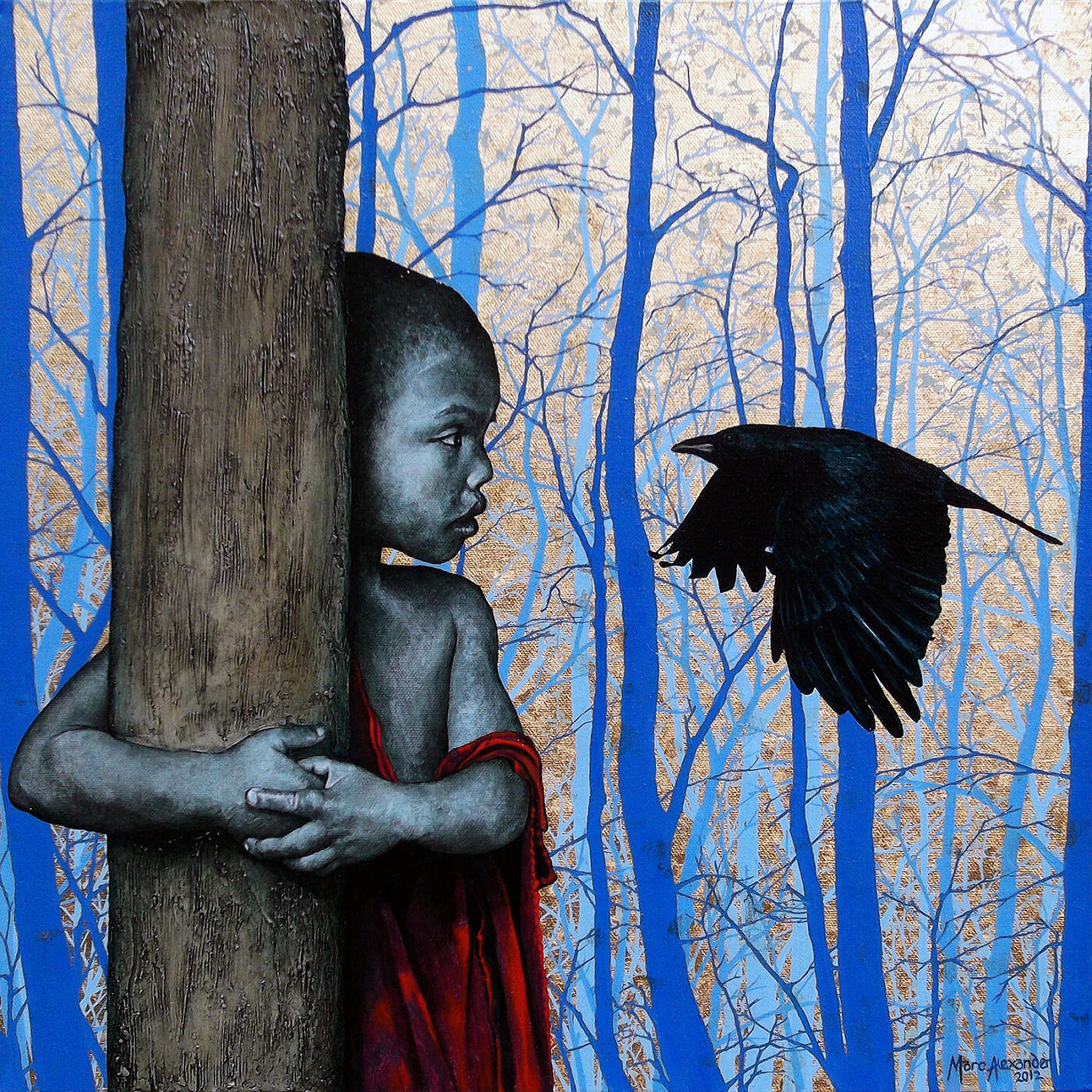##### Enchanted Forest I
Currency conversion from USD to: [convert number=1450.00 from="usd" to="zar"] ZAR | [convert number=1450.00 from="usd" to="gbp"] GBP | [convert number=1450.00 from="usd" to="eur"] EUR     Medium: Oil Gold Leaf on Canvas Size: 50cm by 50cm SOLD
\$ 1,450.00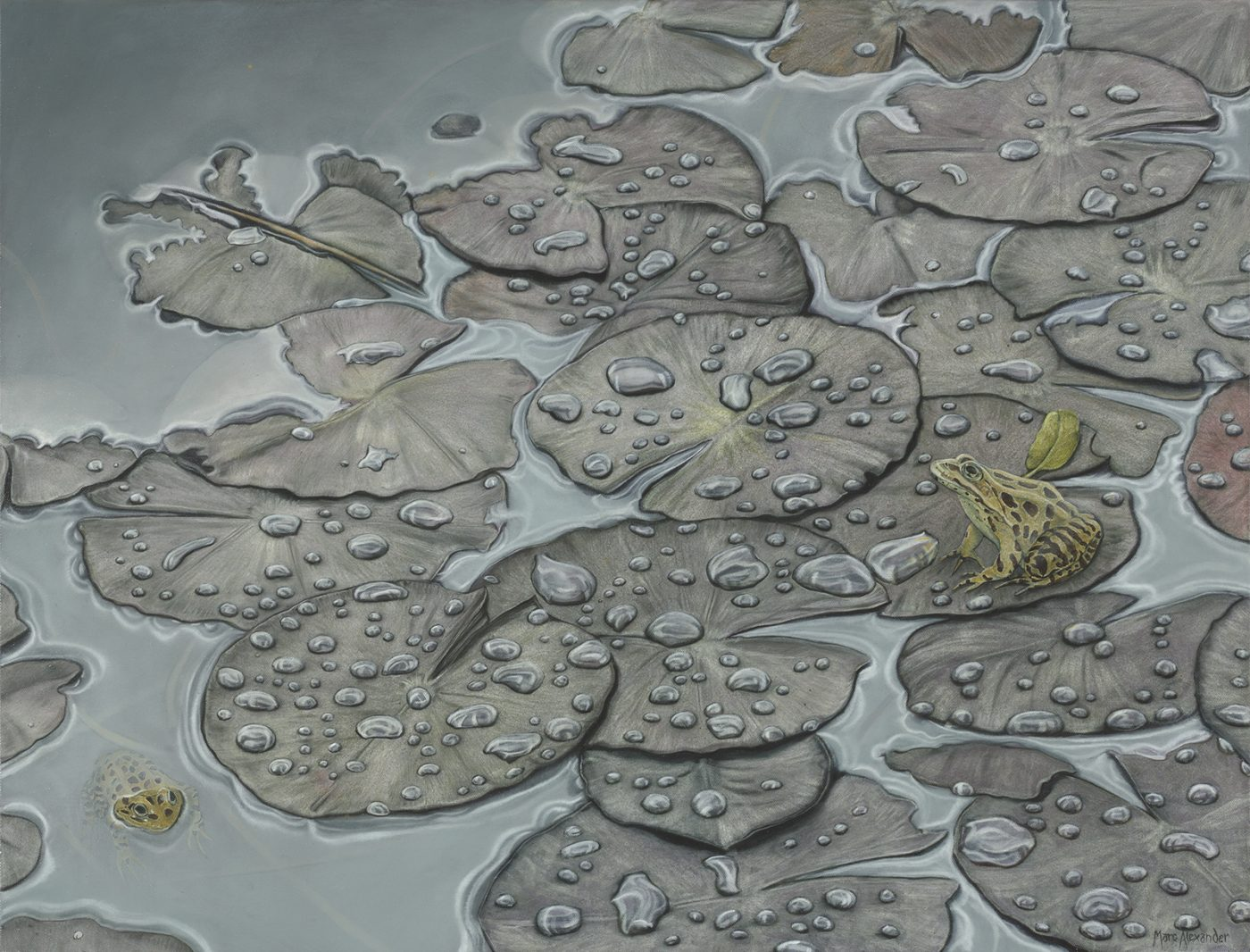Currency conversion from USD to: [convert number=1490.00 from="usd" to="zar"] ZAR | [convert number=1490.00 from="usd" to="gbp"] GBP | [convert number=1490.00 from="usd" to="eur"] EUR   Medium: Oil on Canvas Size: 68cm by 88cm SOLD
\$ 1,490.00##### Aloe I
Currency conversion from USD to: [convert number=2200.00 from="usd" to="zar"] ZAR | [convert number=2200.00 from="usd" to="gbp"] GBP | [convert number=2200.00 from="usd" to="eur"] EUR     Medium: Oil on Canvas Size: 80cm by 140cm SOLD
\$ 2,200.00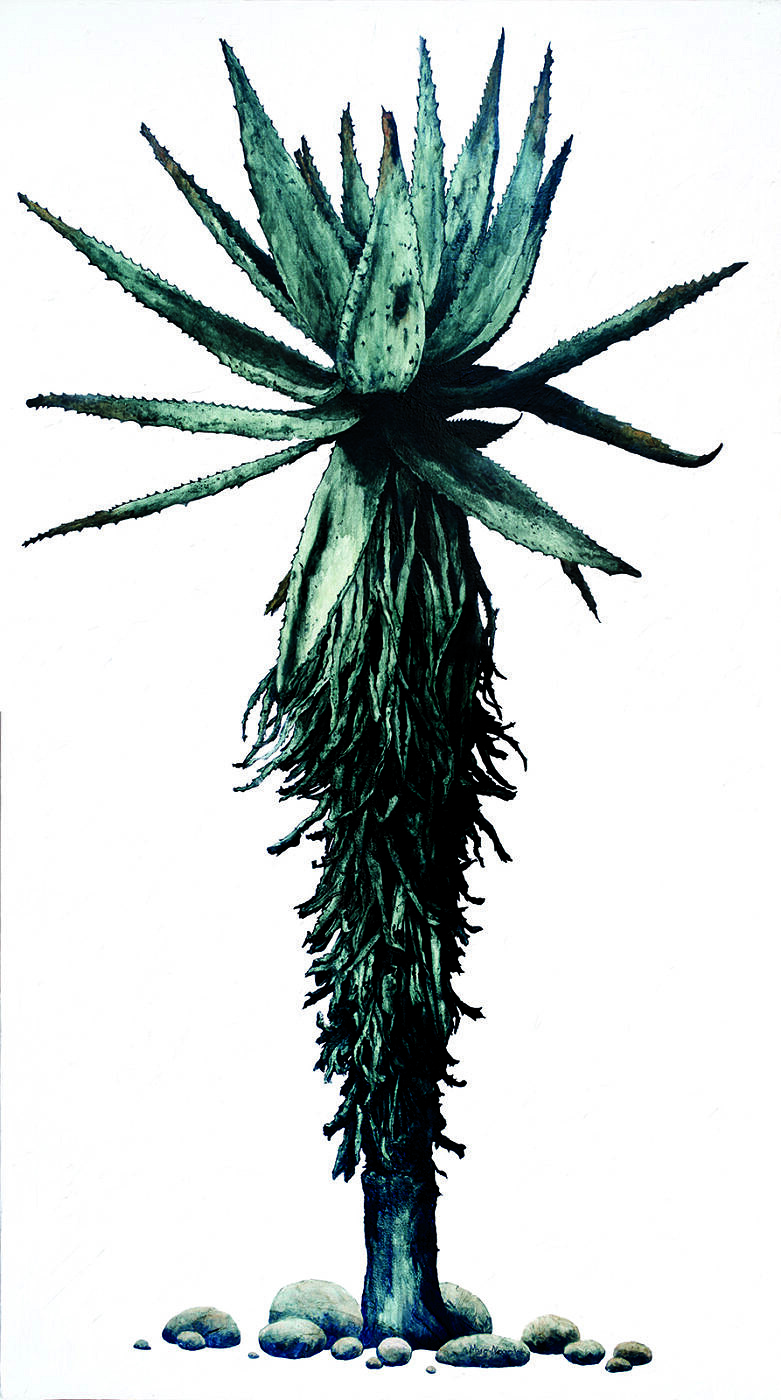##### Aloe III
Currency conversion from USD to: [convert number=2200.00 from="usd" to="zar"] ZAR | [convert number=2200.00 from="usd" to="gbp"] GBP | [convert number=2200.00 from="usd" to="eur"] EUR     Medium: Oil on Canvas Size: 80cm by 140cm SOLD
\$ 2,200.00
Sale!##### Enchanted Forest III
Currency conversion from USD to: [convert number=2200.00 from="usd" to="zar"] ZAR | [convert number=2200.00 from="usd" to="gbp"] GBP | [convert number=2200.00 from="usd" to="eur"] EUR     Medium: Oil and Gold Leaf on Canvas Size: 90cm by 120cm
\$ 3,950.00 \$ 2,200.00##### Himba Children
Currency conversion from USD to: [convert number=2200.00 from="usd" to="zar"] ZAR | [convert number=2200.00 from="usd" to="gbp"] GBP | [convert number=2200.00 from="usd" to="eur"] EUR     Medium: Oil and Gold Leaf on Canvas Size: 90cm by 120cm SOLD
\$ 2,200.00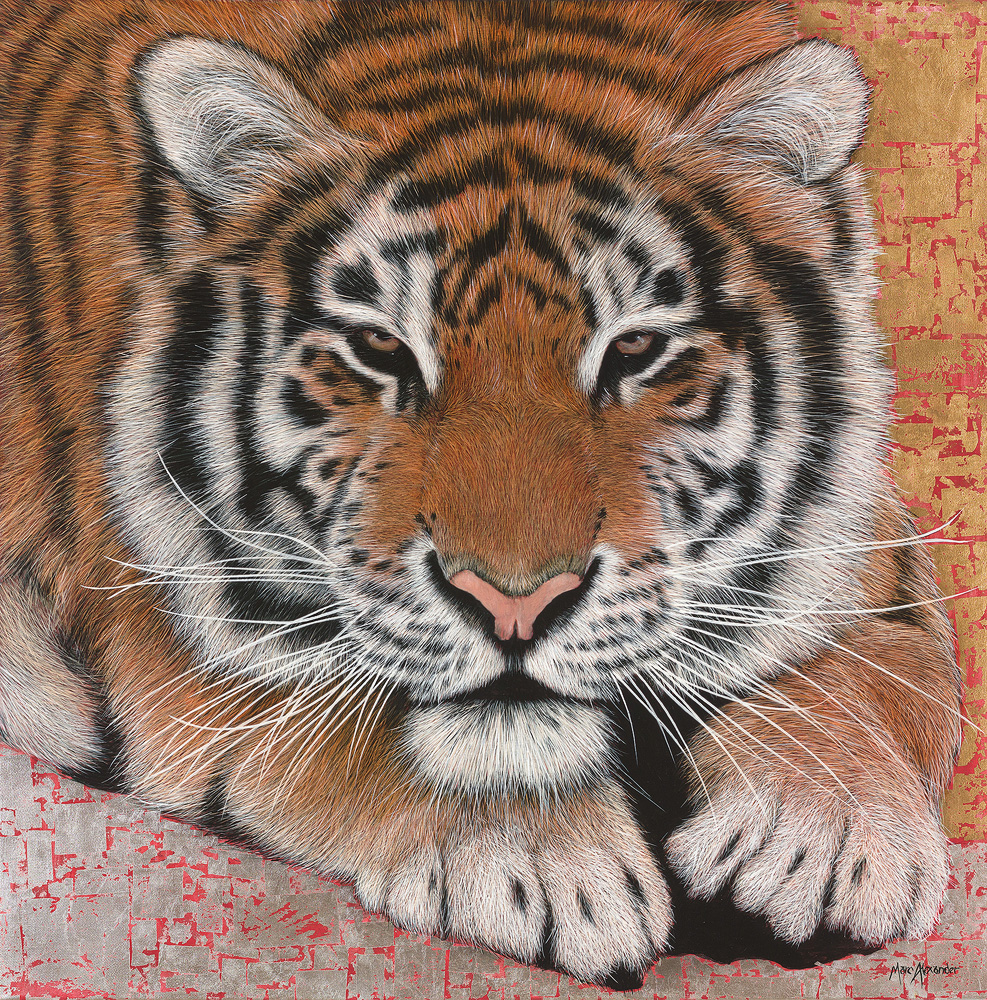##### Siberian Tiger
Currency conversion from USD to: [convert number=2250.00 from="usd" to="zar"] ZAR | [convert number=2250.00 from="usd" to="gbp"] GBP | [convert number=2250.00 from="usd" to="eur"] EUR     Medium: Oil and Silver leaf on Canvas Size: 90cm by 90cm SOLD
\$ 2,250.00##### Giraffe
Currency conversion from USD to: [convert number=2300.00 from="usd" to="zar"] ZAR | [convert number=2300.00 from="usd" to="gbp"] GBP | [convert number=2300.00 from="usd" to="eur"] EUR     Medium: Oil and Gold Leaf on Canvas Size: 100cm by 100cm SOLD
\$ 2,300.00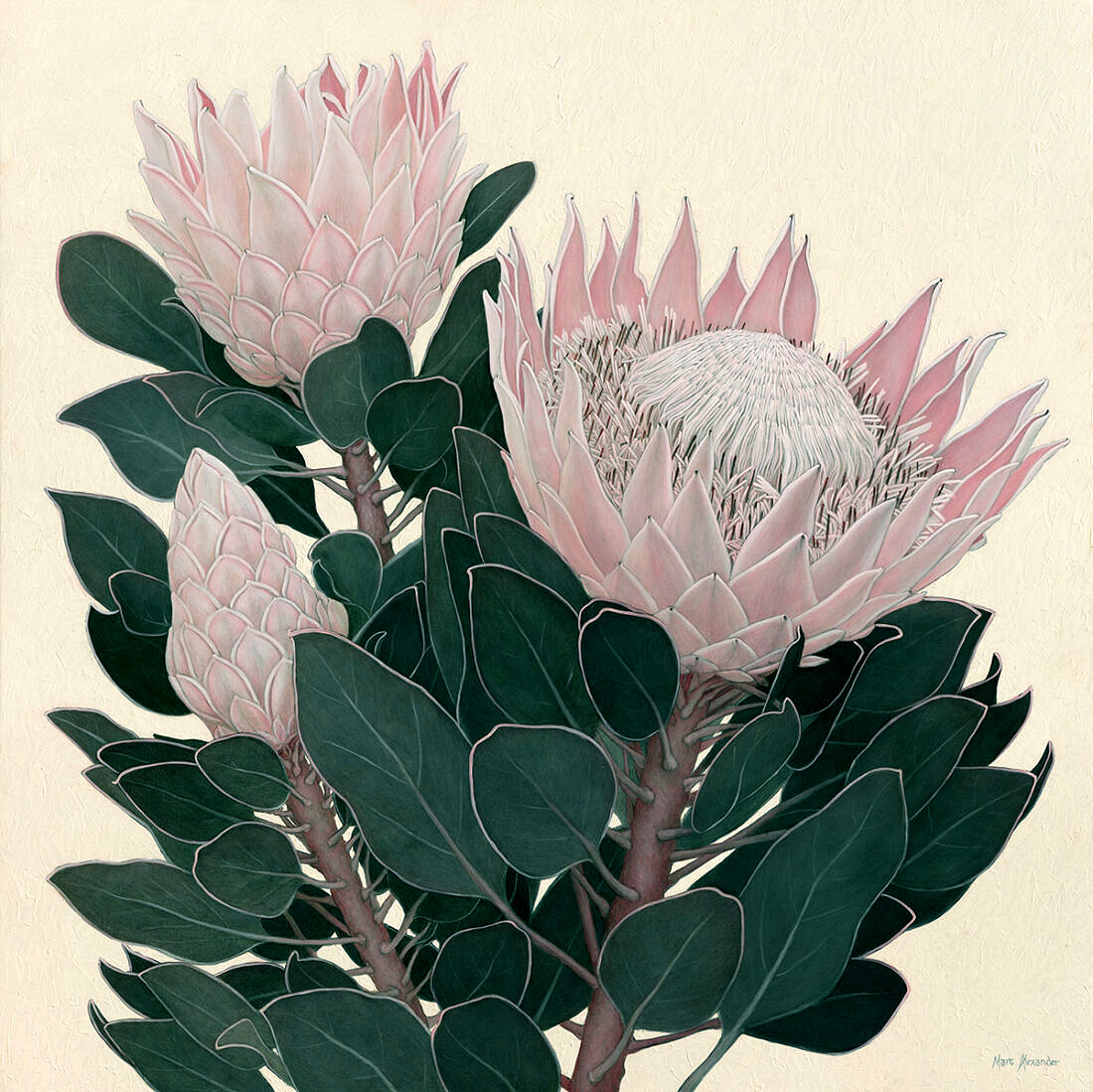##### King Protea I
Currency conversion from USD to: [convert number=2300.00 from="usd" to="zar"] ZAR | [convert number=2300.00 from="usd" to="gbp"] GBP | [convert number=2300.00 from="usd" to="eur"] EUR     Medium: Oil on Canvas Size: 100cm by 100cm SOLD
\$ 2,300.00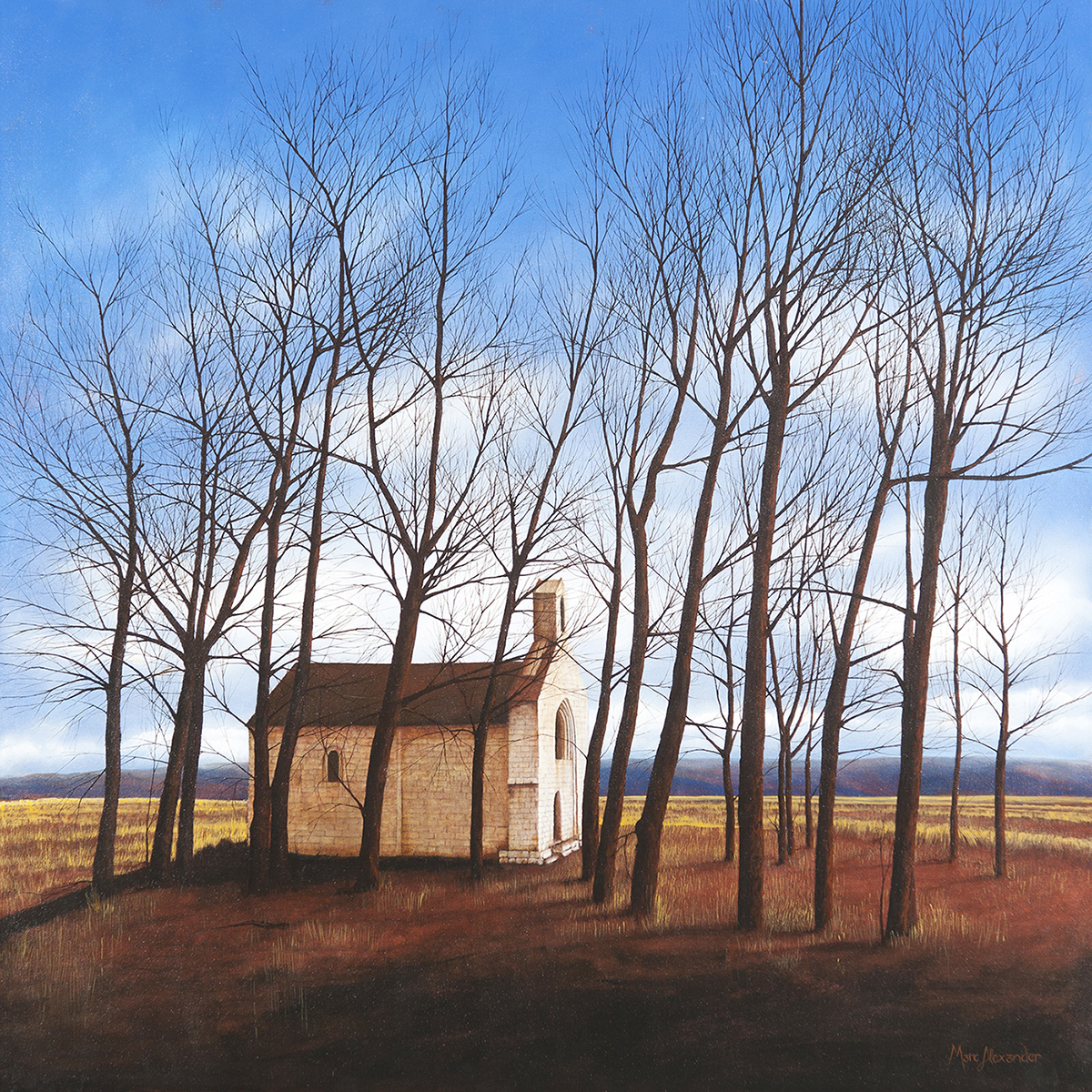##### Winter
Currency conversion from USD to: [convert number=2500.00 from="usd" to="zar"] ZAR | [convert number=2500.00 from="usd" to="gbp"] GBP | [convert number=2500.00 from="usd" to="eur"] EUR     Medium: Oil on Canvas Size: 100cm by 100cm SOLD
\$ 2,500.00##### Spring
Currency conversion from USD to: [convert number=2500.00 from="usd" to="zar"] ZAR | [convert number=2500.00 from="usd" to="gbp"] GBP | [convert number=2500.00 from="usd" to="eur"] EUR     Medium: Oil on Canvas Size: 100cm by 100cm SOLD
\$ 2,500.00##### Enchanted Forest II
Currency conversion from USD to: [convert number=2500.00 from="usd" to="zar"] ZAR | [convert number=2500.00 from="usd" to="gbp"] GBP | [convert number=2500.00 from="usd" to="eur"] EUR     Medium: Oil on Canvas Size: 100cm by 100cm SOLD
\$ 2,500.00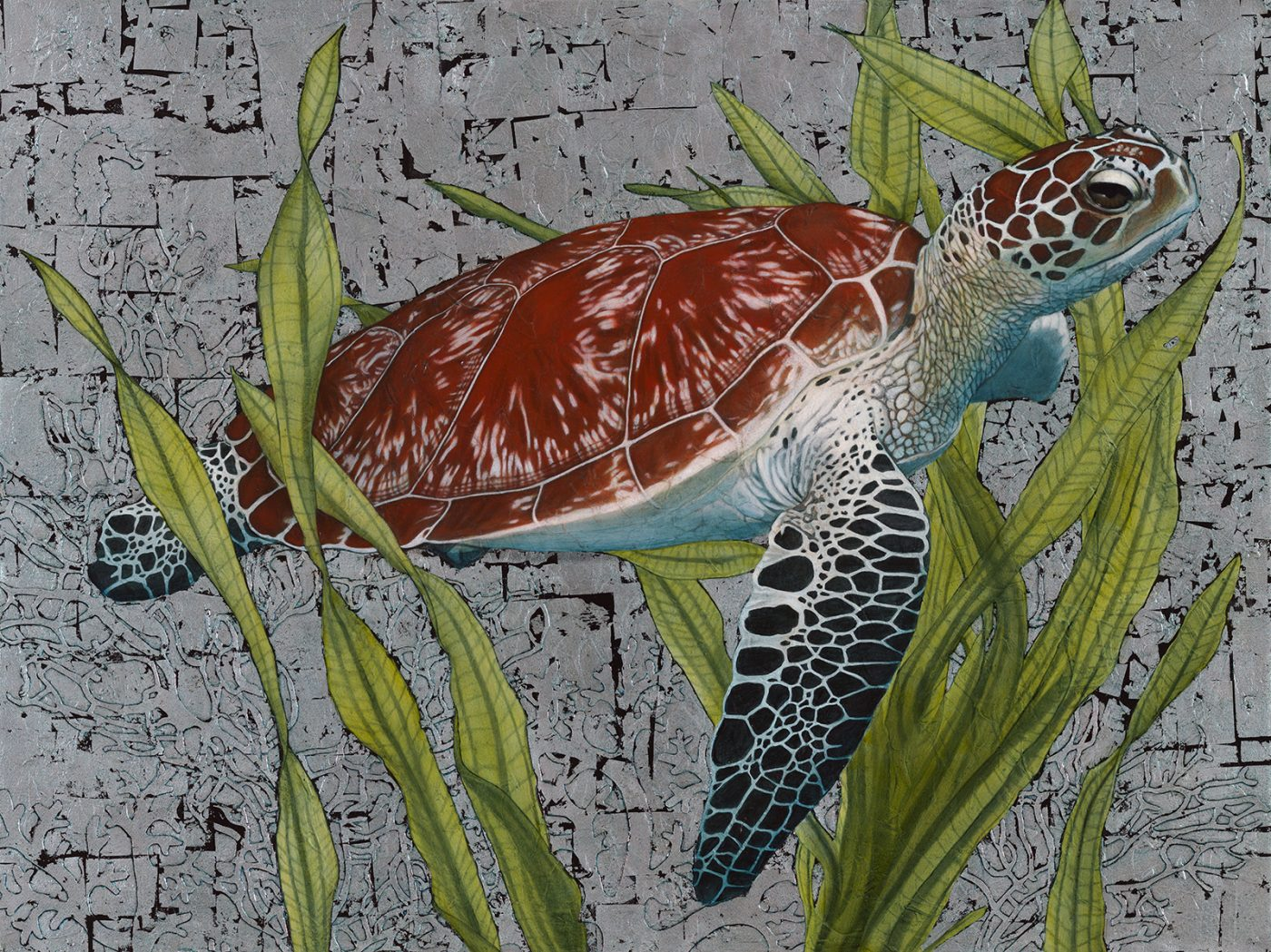##### Hawksbill Sea Turtle
Currency conversion from USD to: [convert number=2550.00 from="usd" to="zar"] ZAR | [convert number=2550.00 from="usd" to="gbp"] GBP | [convert number=2550.00 from="usd" to="eur"] EUR   Medium: Oil and Silver Leaf on Canvas Size: 90cm by 120cm SOLD
\$ 2,550.00##### Bateleur Eagle I
Currency conversion from USD to: [convert number=3450.00 from="usd" to="zar"] ZAR | [convert number=3450.00 from="usd" to="gbp"] GBP | [convert number=3450.00 from="usd" to="eur"] EUR     Medium: Oil and Gold Leaf on Canvas Size: 90cm by 120cm SOLD
\$ 3,450.00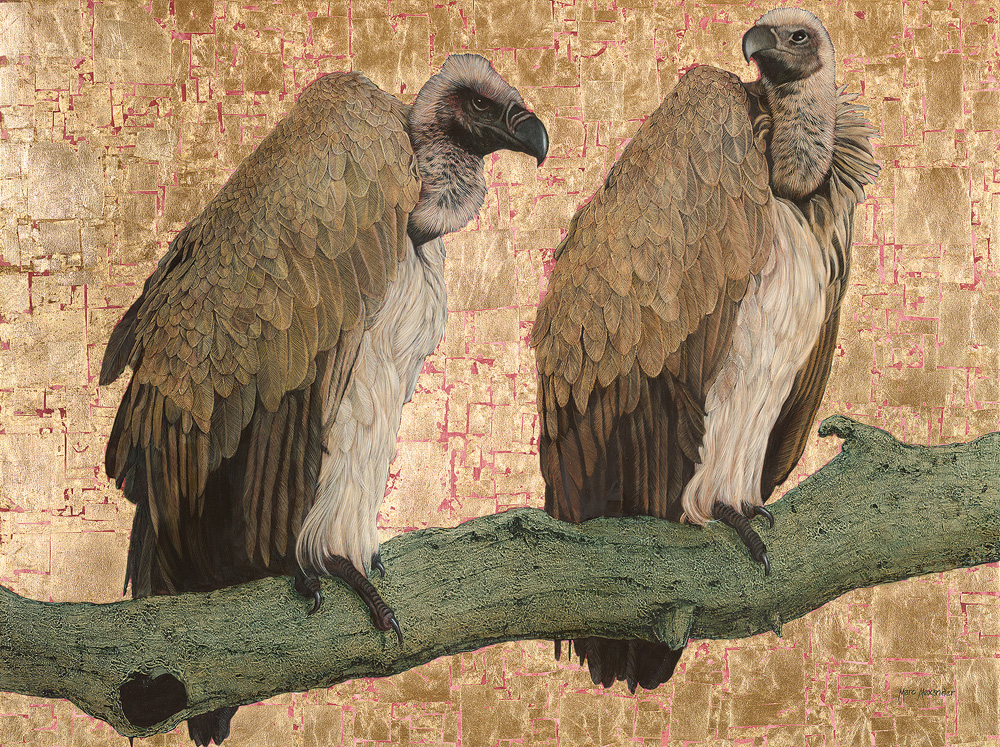##### Cape Vultures
Currency conversion from USD to: [convert number=3650.00 from="usd" to="zar"] ZAR | [convert number=3650.00 from="usd" to="gbp"] GBP | [convert number=3650.00 from="usd" to="eur"] EUR     Medium: Oil and Gold Leaf on Canvas Size: 90cm by 120cm SOLD
\$ 3,650.00##### Rhino II
Currency conversion from USD to: [convert number=3650.00 from="usd" to="zar"] ZAR | [convert number=3650.00 from="usd" to="gbp"] GBP | [convert number=3650.00 from="usd" to="eur"] EUR     Medium: Oil and Gold Leaf on Canvas Size: 90cm by 120cm SOLD
\$ 3,650.00##### Mighty Tusker
Currency conversion from USD to: [convert number=3650.00 from="usd" to="zar"] ZAR | [convert number=3650.00 from="usd" to="gbp"] GBP | [convert number=3650.00 from="usd" to="eur"] EUR     Medium: Oil and Gold Leaf on Canvas Size: 90cm by 120cm SOLD
\$ 3,650.00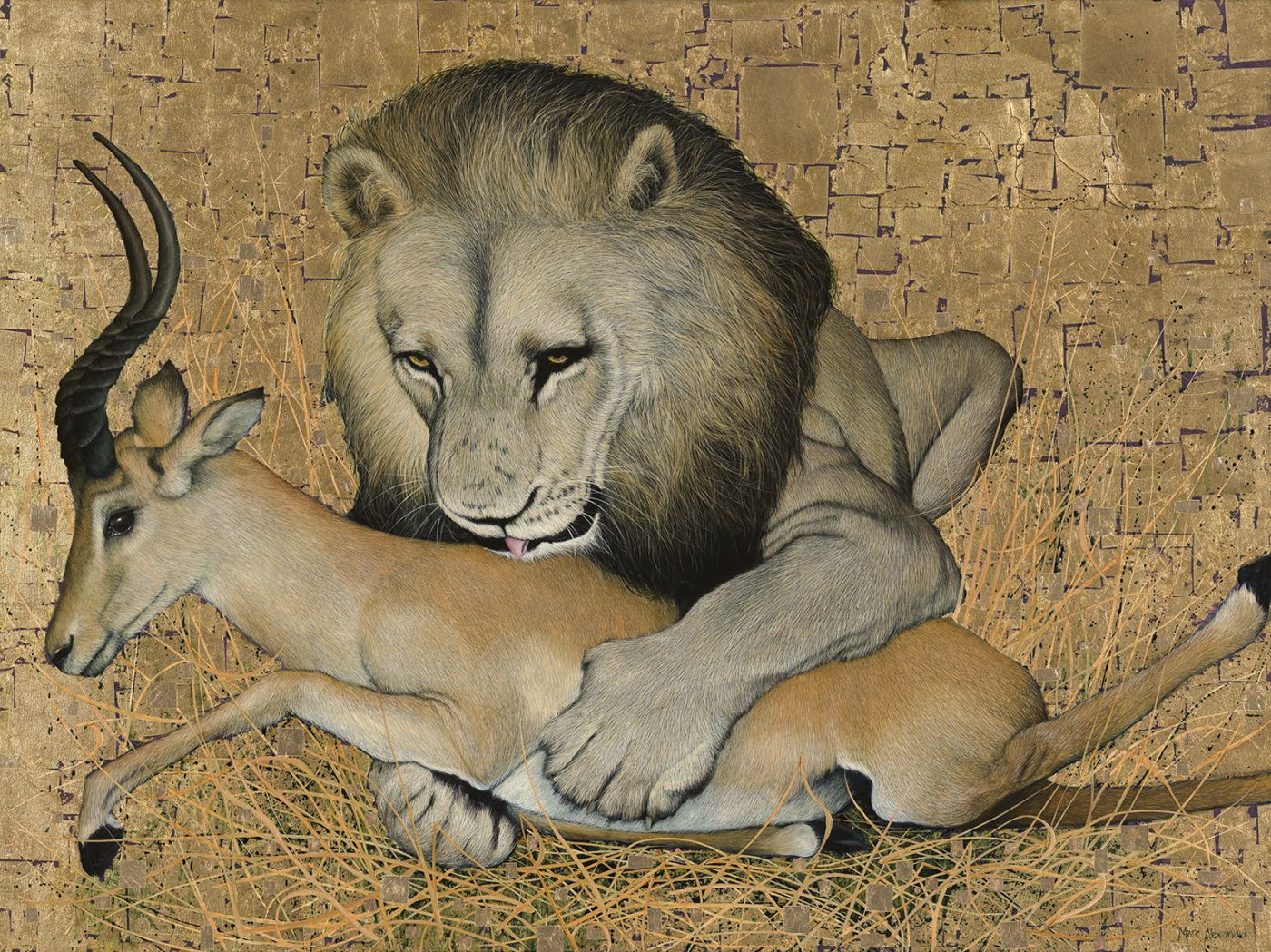##### The Kill
Currency conversion from USD to: [convert number=3650.00 from="usd" to="zar"] ZAR | [convert number=3650.00 from="usd" to="gbp"] GBP | [convert number=3650.00 from="usd" to="eur"] EUR     Medium: Oil and Gold Leaf on Canvas Size: 90cm by 120cm SOLD
\$ 3,650.00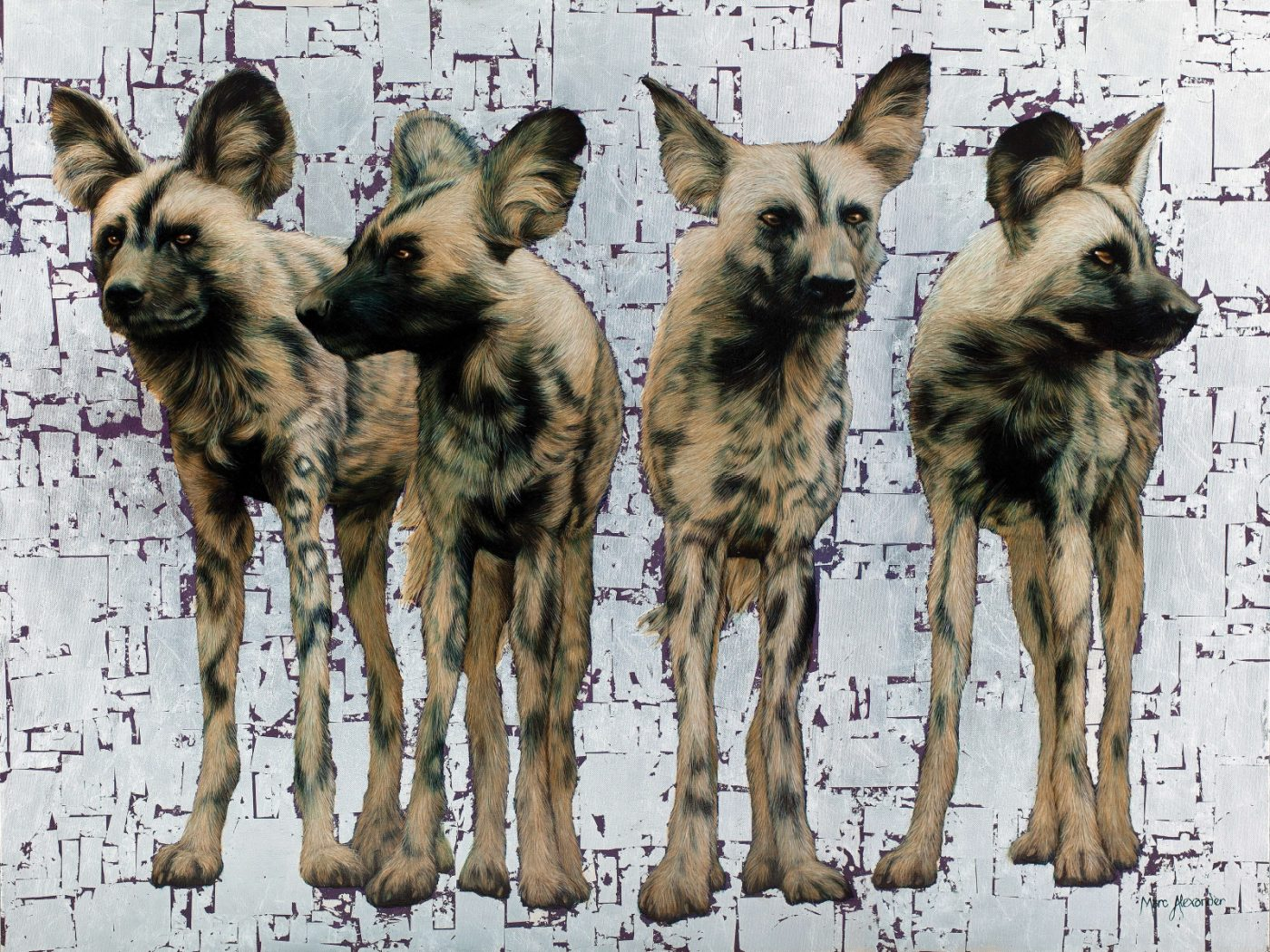##### Wild Dogs Pack of 4
Currency conversion from USD to: [convert number=3650.00 from="usd" to="zar"] ZAR | [convert number=3650.00 from="usd" to="gbp"] GBP | [convert number=3650.00 from="usd" to="eur"] EUR     Medium: Oil and Silver Leaf on Canvas Size: 90cm by 120cm SOLD
\$ 3,650.00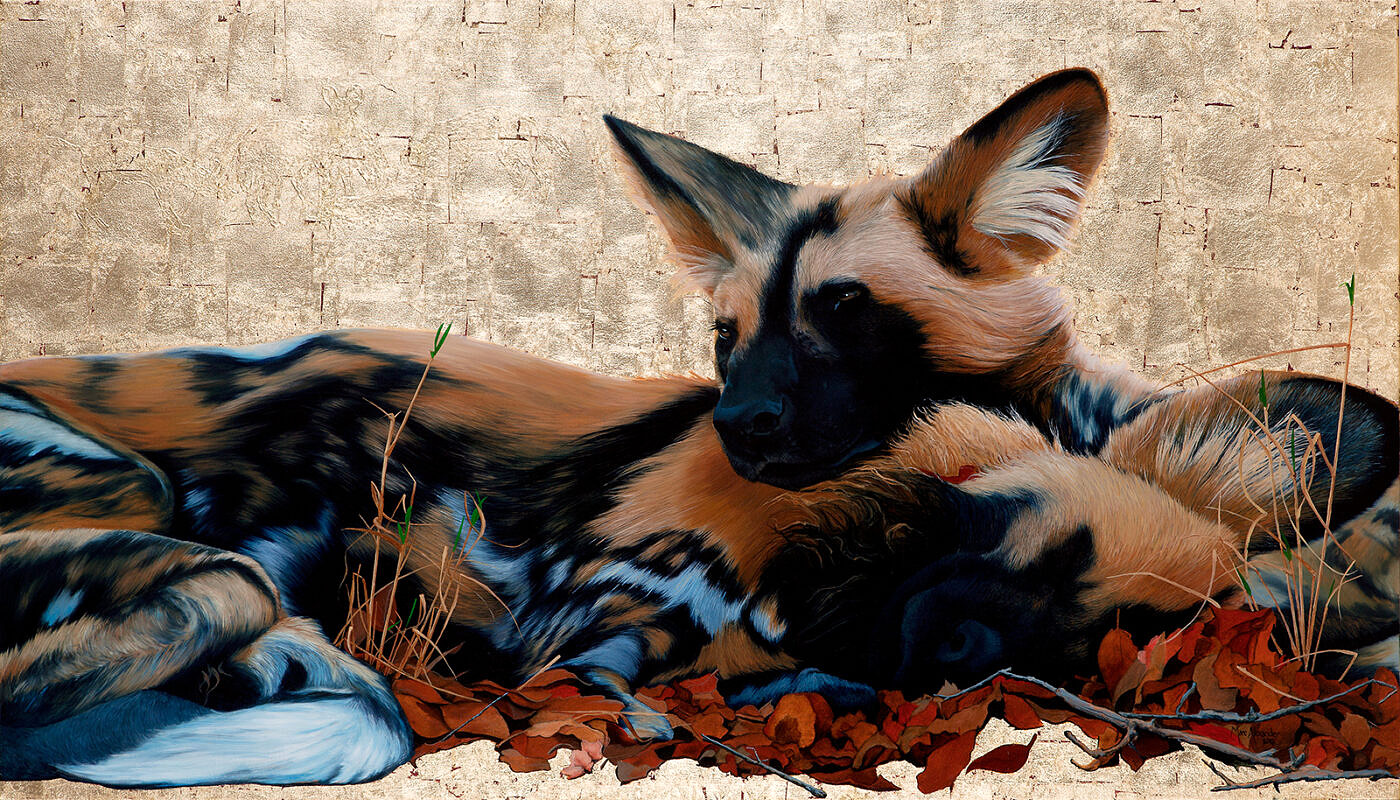##### Wild Dogs I
Currency conversion from USD to: [convert number=3650.00 from="usd" to="zar"] ZAR | [convert number=3650.00 from="usd" to="gbp"] GBP | [convert number=3650.00 from="usd" to="eur"] EUR     Medium: Oil and Gold Leaf on Canvas Size: 80cm by 140cm SOLD
\$ 3,650.00##### Wild Dogs at Dawn
Currency conversion from USD to: [convert number=3650.00 from="usd" to="zar"] ZAR | [convert number=3650.00 from="usd" to="gbp"] GBP | [convert number=3650.00 from="usd" to="eur"] EUR     Medium: Oil and Gold Leaf on Canvas Size: 80cm by 140cm SOLD
\$ 3,650.00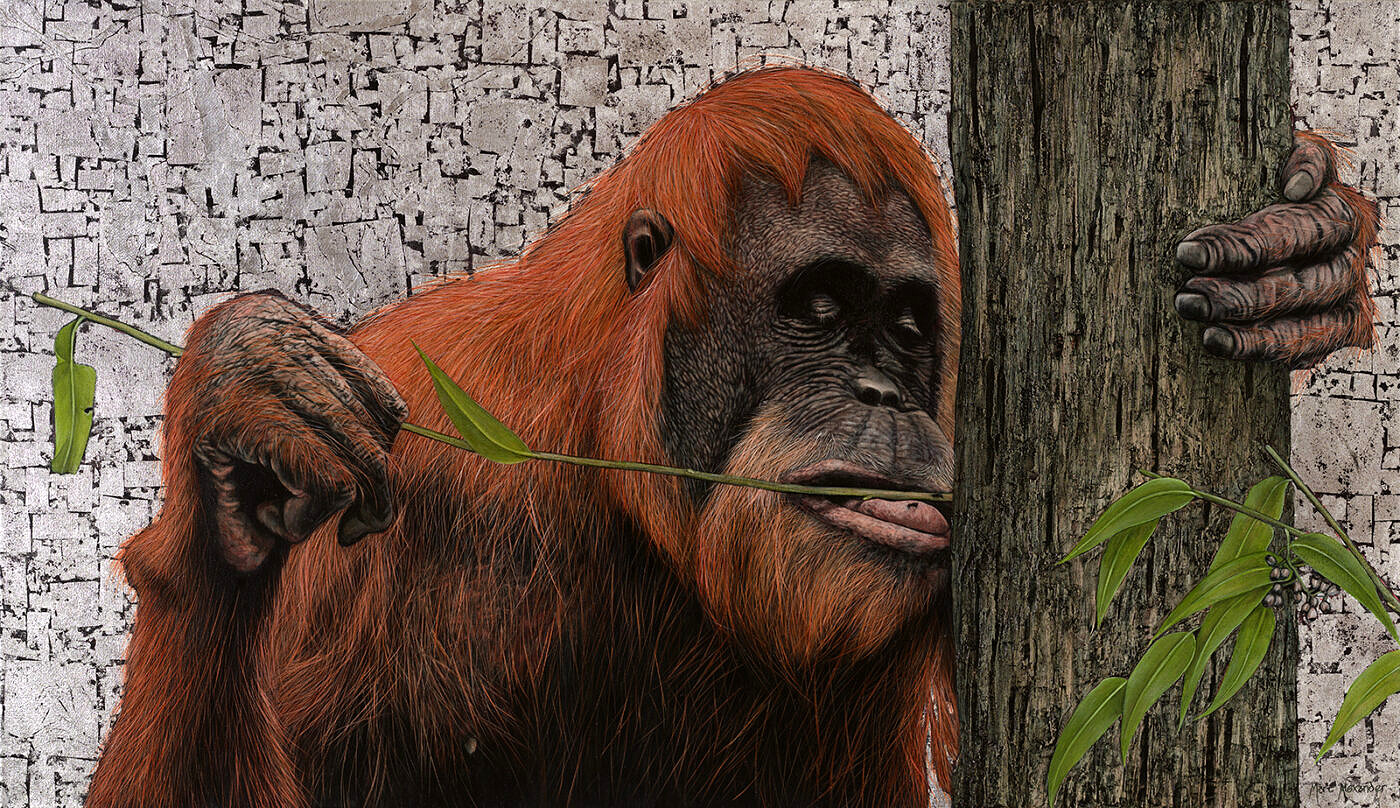##### Sumatran Orangutan
Currency conversion from USD to: [convert number=3650.00 from="usd" to="zar"] ZAR | [convert number=3650.00 from="usd" to="gbp"] GBP | [convert number=3650.00 from="usd" to="eur"] EUR     Medium: Oil and Silver Leaf on Canvas Size: 80cm by 140cm SOLD
\$ 3,650.00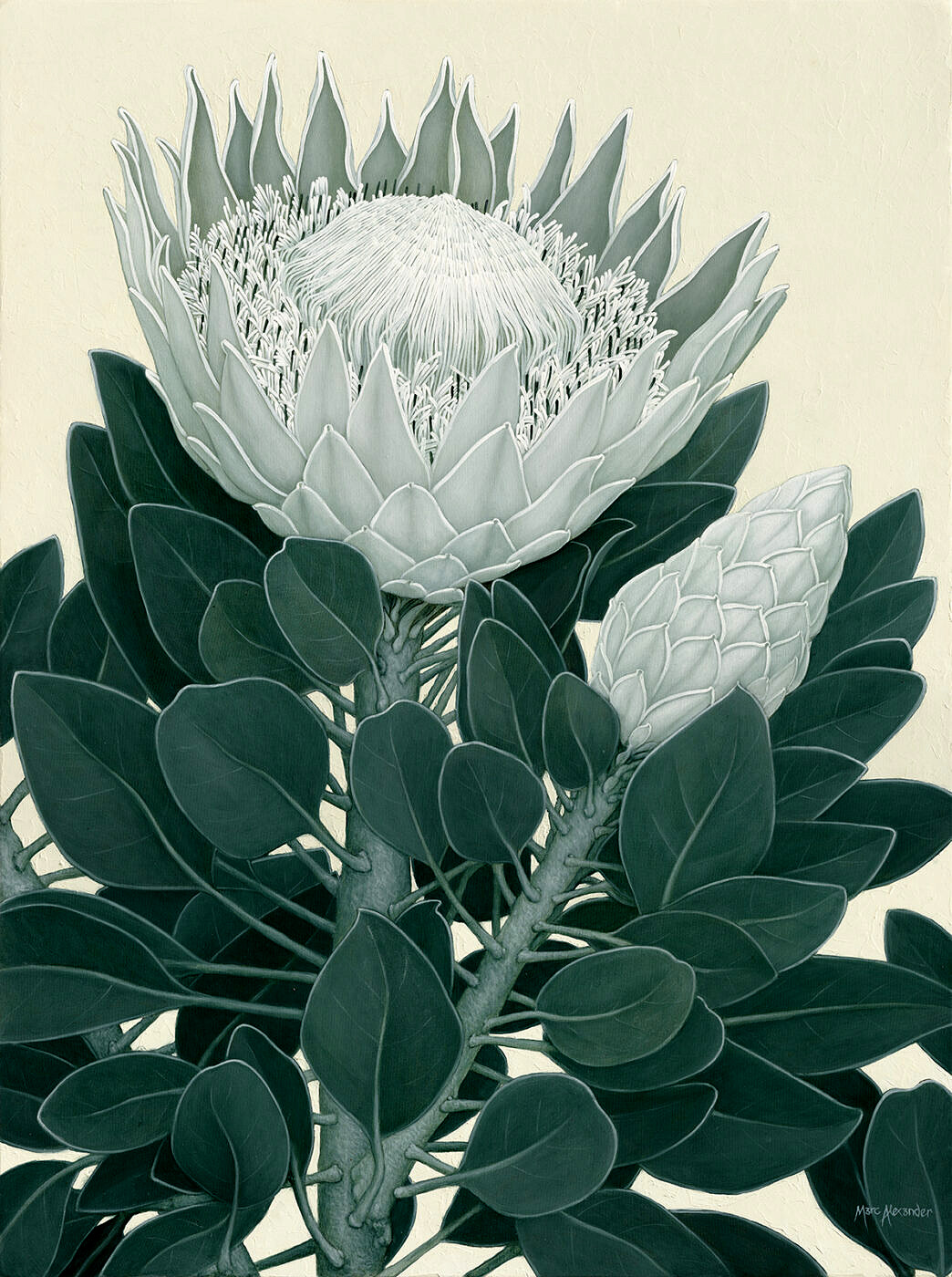##### King Protea II
Currency conversion from USD to: [convert number=3650.00 from="usd" to="zar"] ZAR | [convert number=3650.00 from="usd" to="gbp"] GBP | [convert number=3650.00 from="usd" to="eur"] EUR     Medium: Oil on Canvas Size: 1200cm by 90cm SOLD
\$ 3,650.00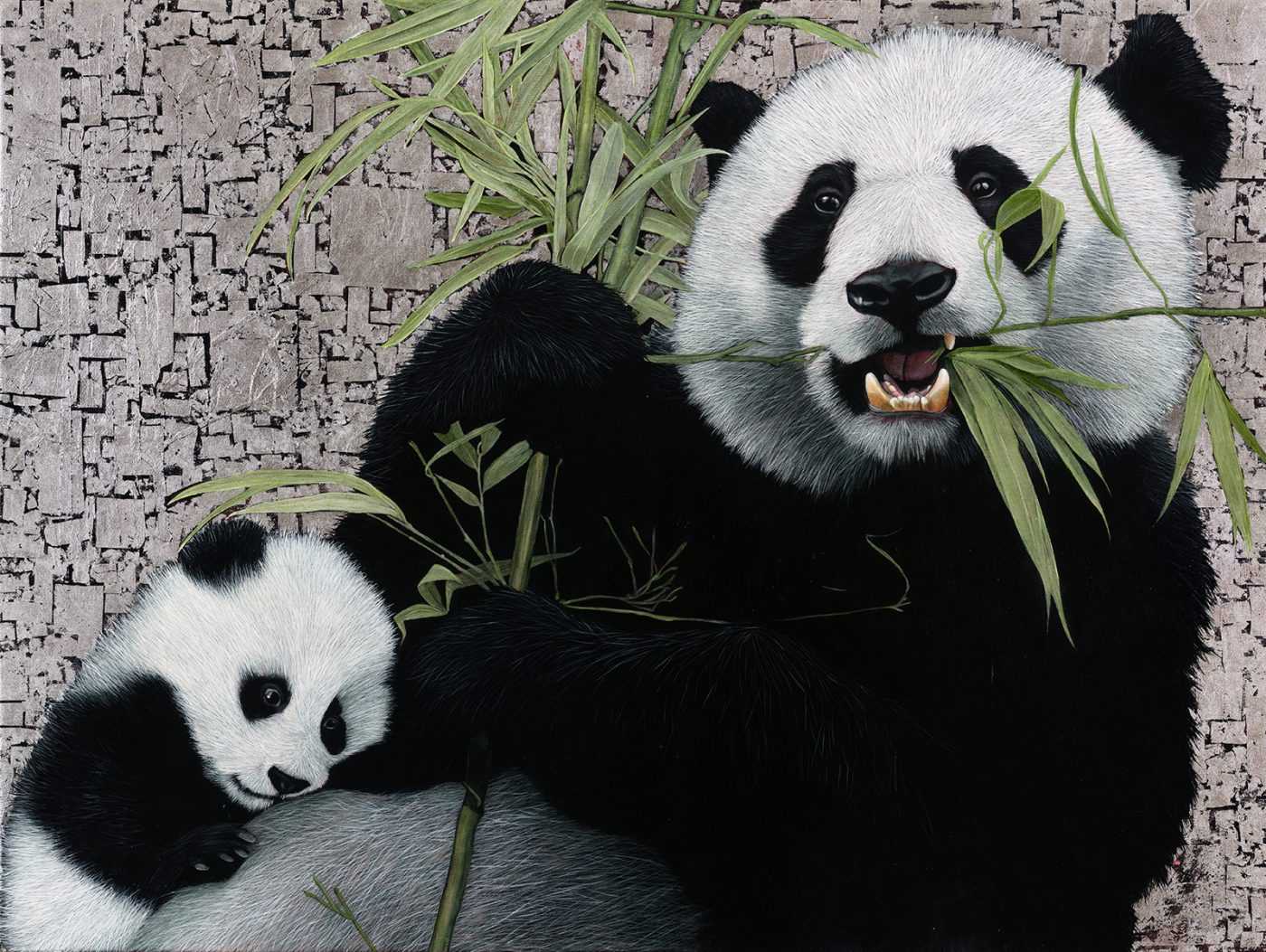##### Giant Pandas
Currency conversion from USD to: [convert number=3700.00 from="usd" to="zar"] ZAR | [convert number=3700.00 from="usd" to="gbp"] GBP | [convert number=3700.00 from="usd" to="eur"] EUR   Medium: Oil and Silver Leaf on Canvas Size: 90cm by 120cm SOLD
\$ 3,700.00##### Bateleur Eagle II
Currency conversion from USD to: [convert number=3850.00 from="usd" to="zar"] ZAR | [convert number=3850.00 from="usd" to="gbp"] GBP | [convert number=3850.00 from="usd" to="eur"] EUR     Medium: Oil and Gold Leaf on Canvas Size: 120cm by 90cm SOLD
\$ 3,850.00##### African Buffalo
Currency conversion from USD to: [convert number=3450.00 from="usd" to="zar"] ZAR | [convert number=3450.00 from="usd" to="gbp"] GBP | [convert number=3450.00 from="usd" to="eur"] EUR     Medium: Oil and Gold Leaf on Canvas Size: 90cm by 120cm SOLD
\$ 3,975.00